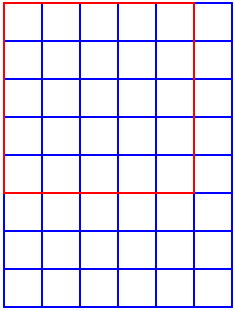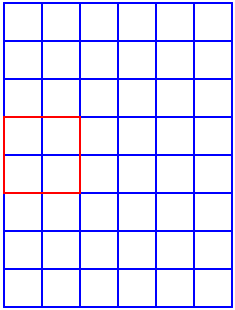#### You may also like### Square Areas

Can you work out the area of the inner square and give an explanation of how you did it?### Dissect

What is the minimum number of squares a 13 by 13 square can be dissected into?### 2001 Spatial Oddity

With one cut a piece of card 16 cm by 9 cm can be made into two pieces which can be rearranged to form a square 12 cm by 12 cm. Explain how this can be done.

# Squares in Rectangles

##### Age 11 to 14Challenge Level

Many people suggested the answer should be $6$ by $8$, because this is "double" the $6$ by $4$ example given in the problem. However, this doesn't take into account squares contained in the $6$ by $8$ rectangle which are not contained in the original $6$ by $4$ rectangle, like the red ones below:In the first picture, we have a $5 \times 5$ square. You cannot fit any of these in a $6 \times 4$ rectangle, but you can fit them in a $6 \times 8$ rectangle.

Imagine the $6 \times 8$ rectangle is made up from joining two $6 \times 4$ rectangles. In the second picture, you can see a $2 \times 2$ square. Where the $6 \times 4$ rectangles meet there will be an extra row of these $2 \times 2$ squares that would not be in either of the $6 \times 4$ rectangles.

One way to approach this problem is to try some examples and set your working out in a table, and then look for patterns and try to explain them. Sam from OPGS sent us his table:

 columns: rows: 1 2 3 4 5 6 7 1 1 2 3 4 5 6 7 2 2 5 8 11 14 17 20 3 3 8 14 20 26 32 38 4 4 11 20 30 40 50 60 5 5 14 26 40 55 70 85 6 6 17 32 50 70 91 112 7 7 20 38 60 85 112 140

Since the number of squares in an $n \times m$ rectangle is the same as the number of squares in an $m \times n$ rectangle, we will look only at rectangles with at least as many columns as rows. These are italicised in the above table.

First we notice that, if there is one row, increasing the number of columns by one increases the number of squares by $1$. This is because the only size of square that we can make is $1 \times 1$, so adding a column adds just one square.

If there are two rows, adding an extra column increases the number of squares by $3$. Why is this? Well, if we add an extra column we can make $2$ additional $1 \times 1$ squares and $1$ additional $2 \times 2$ square. This is a total of three extra squares. If we continue the pattern $2, 5, 8, \ldots$ in the $2$ row, we eventually get to $\ldots, 98, 101, \ldots$ - missing out $100$. This tells us that it isn't possible to make a rectangle with $100$ squares which has $2$ rows.

If there are three rows, adding an extra column allows us to make 3 more $1 \times 1$ squares, $2$ more $2 \times 2$ squares and $1$ more $3 \times 3$ square. This is a total of $6 = 3 + 2 + 1$ squares. This gives us the sequence $14, 20, 26, 32, \ldots, 98, 104, \ldots$ which tells us that we can't make a rectangle with exactly $100$ squares which has $3$ rows.

Using the same reasoning for four rows, we see adding a column increases the number of squares by $10$, and that we can make rectangles with $20, 30, \ldots, 90, 100, 110, \ldots$ squares. $100$ is in this list! In fact, a $4 \times 11$ rectangle contains exactly $100$ squares.

We can repeat this for the other rows in the table to find all the rectangles with exactly $100$ squares. These are $1 \times 100$, $4 \times 11$ and $5 \times 8$.

Mr Lunn's Year 7 class from The Wensleydale School reasoned in a very similar way. Here is a summary of their findings, showing a link between the results and Triangle Numbers.

Sandeep from Nrayana Junior College and Terence from Brumby Engineering College used a slightly different approach to count the number of squares in rectangles of different sizes, starting with the examples from the problem page. Here is Sandeep's method:

In a $2 \times 3$ rectangle, squares of side $1$ unit and squares of side $2$ units can be formed.

Number of squares of side $1$ unit is $2 \times 3 = 6$.
Number of squares of side $2$ units is $1 \times 2 = 2$.
Therefore the total number of squares in a $2 \times 3$ rectangle is $6 + 2 = 8$.

Similarly if we consider a $3 \times 4$ rectangle there are a total of $20$ squares.

Number of squares of side $1$ unit: $3 \times 4 = 12$.
Number of squares of side $2$ units: $2 \times 3 = (3-1) \times (4-1) = 6$.
Number of squares of side $3$ units: $1 \times 2 = (3-2) \times (4-2) = 2$.
Total number of squares $= 12 + 6 + 2 = 20$.

We can generalise this fact by taking a rectangle of $x$ rows and $y$ columns: $$\text{Total number of squares} = x \times y + (x-1) \times (y-1) + (x-2) \times (y-2) + \ldots$$ The addition series stops only when either the rows or the columns reduces to 1.

By trial and error, 5 $\times$ 8 and 4 $\times$ 11 rectangles contain exactly 100 squares. There can be no other rectangles containing 100 squares. This can be verified by increasing or decreasing the number of rows and columns.